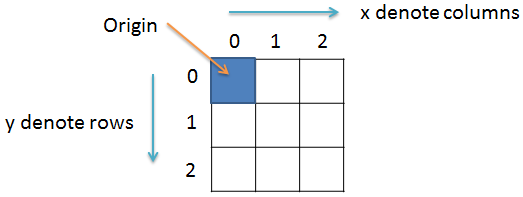# How to create a mirror image in Java

Image Processing Project

In this project we will learn to create a mirror image using Java programming language.

### Prerequisite

It is assumed that you have completed the projects titled How to read and write image file in Java and How to get and set pixel value in Java before starting this project.

### Mirror image

To create a mirror image we have to build the image pixel by pixel.

1. Read the source image in BufferedImage object simg.
2. Get the dimension of the source image.
3. Create a BufferedImage object mimg for mirror image. Set the width of mimg to twice the original image (simg) width.
4. Copy source image pixel by pixel to destination image.

### Import the required classes

Create a new file and save it by the name MirrorImage.java. Open the file and import the following:

``import java.io.File;``import java.io.IOException;``import java.awt.image.BufferedImage;``import javax.imageio.ImageIO;``

### Create the class

Next we will create the class by the name MirrorImage and inside the class we will have the main() method. For this we will write:

``public class MirrorImage{``  public static void main(String args[])throws IOException{``  // some code goes here...``  }//main() ends here``}//class ends here``

### Variables

Inside the main() method we create a BufferedImage variable simg and a File variable f and set both of them to null. The simg variable will hold the source image file while the f variable will hold the image file path.

`BufferedImage simg = null;File f = null;`

For this project we will read the image audrey.jpg and it is inside the Image folder which is inside D: drive on a Windows PC. So, we will first create an object of File class and pass as parameter the image file path. And then we will read the image file by calling the read() method of ImageIO class. So, our code will look something like the following:

``//read image``try{``  f = new File("D:\\Image\\audrey.jpg");``  simg = ImageIO.read(f);``}catch(IOException e){``  System.out.println(e);``}``

### Get source image dimensions

Now that we have the source image stored in the simg variable, it's time for us to find its dimensions. For this we will create two integer variable width and height and use the getWidth() and getHeight()method to get the width and height of the source image respectively. So, we will write the following lines.

``//get source image width and height``int width = simg.getWidth();``int height = simg.getHeight();``

### Mirror image variable

Now we will create a BufferedImage object mimg that will store the mirror image. We will set the width of the mirror image to twice the source image. Height of the source image and mirror image will be same. So, for this we will write the following code:

``//BufferedImage for mirror image``BufferedImage mimg = new BufferedImage(width*2, height, BufferedImage.TYPE_INT_ARGB);``

### Mirror image code

We know that an image is made up of pixels which we can represent in 2D co-ordinate. So, we will create three variables, lx, rx and y and use two for loops to traverse each pixels.For this we will write:

``for(int y = 0; y < height; y++){``  for(int lx = 0, rx = width*2 - 1; lx < width; lx++, rx--){    //lx starts from the left side of the image    //rx starts from the right side of the image    // some code goes here...  }}``

### Get source pixel value and set in mirror image

Now, we will get the source pixel value at co-ordinate (x,y) and set it in mirror image ming at co-ordinate (lx, y) and (rx, y) which will give a mirror effect. For this we will write:

``//get source pixel value``int p = simg.getRGB(lx, y);``//set mirror image pixel value - both left and right``mimg.setRGB(lx, y, p);``mimg.setRGB(rx, y, p);``

### Write image

After the for loop ends we will write the image file. For this we will write the following code:

``try{``  f = new File("D:\\Image\\Output.jpg");``  ImageIO.write(img, "png", f);``}catch(IOException e){``  System.out.println(e);``}``

### Final code

``/**`` * File: MirrorImage.java`` * `` * Description:`` * Create a mirror image.`` * `` * @author Yusuf Shakeel`` * Date: 04-04-2014 fri`` */``import java.io.File;``import java.io.IOException;``import java.awt.image.BufferedImage;``import javax.imageio.ImageIO;``public class MirrorImage{``  public static void main(String args[])throws IOException{``    //BufferedImage for source image``    BufferedImage simg = null;``    //File object``    File f = null;``    //read source image file``    try{``      f = new File("D:\\Image\\audrey.jpg");``      simg = ImageIO.read(f);``    }catch(IOException e){``      System.out.println("Error: " + e);``    }``    //get source image dimension``    int width = simg.getWidth();``    int height = simg.getHeight();``    //BufferedImage for mirror image``    BufferedImage mimg = new BufferedImage(width*2, height, BufferedImage.TYPE_INT_ARGB);``    //create mirror image pixel by pixel``    for(int y = 0; y < height; y++){``      for(int lx = 0, rx = width*2 - 1; lx < width; lx++, rx--){``        //lx starts from the left side of the image``        //rx starts from the right side of the image``        //get source pixel value``        int p = simg.getRGB(lx, y);``        //set mirror image pixel value - both left and right``        mimg.setRGB(lx, y, p);``        mimg.setRGB(rx, y, p);``      }``    }``    //save mirror image``    try{``      f = new File("D:\\Image\\Output.png");``      ImageIO.write(mimg, "png", f);``    }catch(IOException e){``      System.out.println("Error: " + e);``    }``  }//main() ends here``}//class ends here``